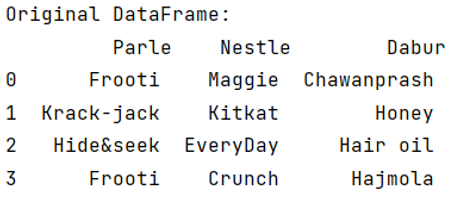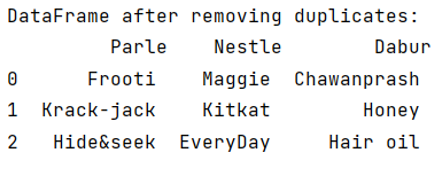# How to remove duplicate columns in Pandas DataFrame?

Given a Pandas DataFrame, we have to remove duplicate columns.
Submitted by Pranit Sharma, on May 28, 2022

Columns are the different fields that contain their particular values when we create a DataFrame. We can perform certain operations on both rows & column values.

Duplicity is a column of pandas DataFrame occurs when there is more than 1 occurrence of similar elements.

Here, we are going to learn how to remove duplicate columns in pandas DataFrame. For this purpose, we are going to use pandas.DataFrame.drop_duplicates() method.

## pandas.DataFrame.drop_duplicates() Method

This method is useful when there are more than 1 occurrence of a single element in a column. It will remove all the occurrences of that element except one.

Syntax:

```DataFrame.drop_duplicates(
subset=None,
keep='first',
inplace=False,
ignore_index=False
)
```

Parameter(s):

• Subset: It takes a list or series to check for duplicates.
• Keep: It is a control technique for duplicates.
• inplace: It is a Boolean type value that will modify the entire row if True.

To work with pandas, we need to import pandas package first, below is the syntax:

```import pandas as pd
```

Let us understand with the help of an example.

```# Importing pandas package
import pandas as pd

# Defining two DataFrames
df = pd.DataFrame(data = {
'Parle':['Frooti','Krack-jack','Hide&seek','Frooti'],
'Nestle':['Maggie','Kitkat','EveryDay','Crunch'],
'Dabur':['Chawanprash','Honey','Hair oil','Hajmola']
})

# Display DataFrame
print("Original DataFrame:\n",df,"\n")
```

Output:Here, we can observe that a column has a duplicate value, so removing these duplicates is necessary.

```# Removing duplicates
result = df.drop_duplicates(subset="Parle")

# Display result
print("DataFrame after removing duplicates:\n",result)
```

Output:Preparation

What's New

Top Interview Coding Problems/Challenges!